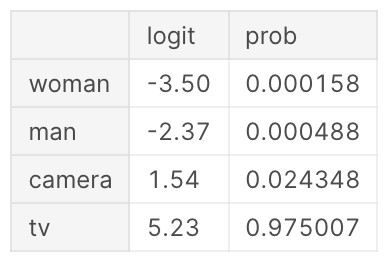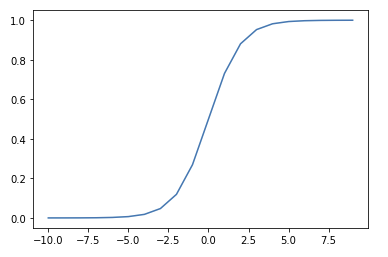• ## Softmax Activation FunctionThe Softmax function converts a vector of numbers into a vector of probabilities that sum to 1. It's applied to a model's outputs (or Logits …

• ## Sigmoid Activation FunctionThe Sigmoid function squeezes numbers into a probability-like range between 0 and 1.1 Used in Binary Classification model architectures to compute loss on discrete …